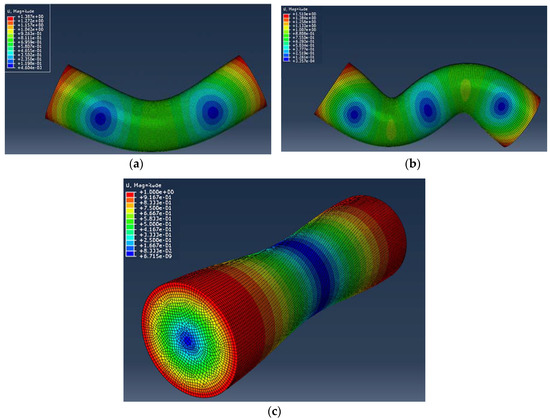Next Article in Journal
Accelerometer Based Method for Tire Load and Slip Angle Estimation
Open AccessArticle

Direct Determination of Dynamic Elastic Modulus and Poisson’s Ratio of Timoshenko Rods

byGuadalupe LeonandHung-Liang (Roger) Chen *Department of Civil and Environmental Engineering, West Virginia University, Morgantown, WV 26506, USA
*
Author to whom correspondence should be addressed.
Vibration 2019, 2(1), 157-173; https://doi.org/10.3390/vibration2010010
Received: 4 December 2018 / Revised: 23 February 2019 / Accepted: 1 March 2019 / Published: 7 March 2019
In this paper, the exact solution of the Timoshenko circular beam vibration frequency equation under free-free boundary conditions was determined with an accurate shear shape factor. The exact solution was compared with a 3-D finite element calculation using the ABAQUS program, and the difference between the exact solution and the 3-D finite element method (FEM) was within 0.15% for both the transverse and torsional modes. Furthermore, relationships between the resonance frequencies and Poisson’s ratio were proposed that can directly determine the elastic constants. The frequency ratio between the 1st bending mode and the 1st torsional mode, or the frequency ratio between the 1st bending mode and the 2nd bending mode for any rod with a length-to-diameter ratio, L/D ≥ 2 can be directly estimated. The proposed equations were used to verify the elastic constants of a steel rod with less than 0.36% error percentage. The transverse and torsional frequencies of concrete, aluminum, and steel rods were tested. Results show that using the equations proposed in this study, the Young’s modulus and Poisson’s ratio of a rod can be determined from the measured frequency ratio quickly and efficiently. View Full-Text
Show FiguresFigure 1

MDPI and ACS Style

Leon, G.; Chen, H.-L.R. Direct Determination of Dynamic Elastic Modulus and Poisson’s Ratio of Timoshenko Rods. Vibration 2019, 2, 157-173.

Show more citation formats Show less citations formats

1